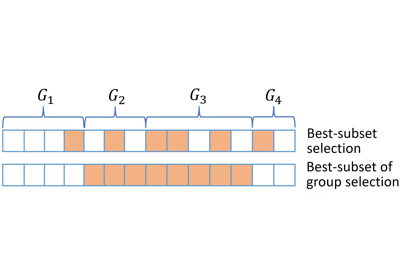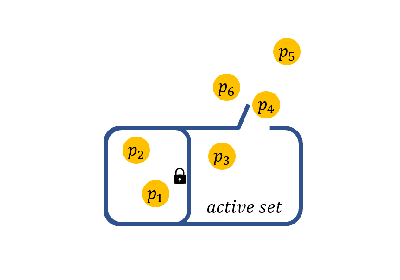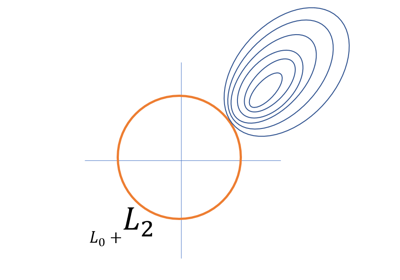When analyzing the real world datasets, we may have the following targets:

1. identifying predictors when group structure are provided (a.k.a., best group subset selection);

2. certain variables must be selected when some prior information is given (a.k.a., nuisance regression);

3. selecting the weak signal variables when the prediction performance is mainly interested (a.k.a., regularized best-subset selection).

These targets are frequently encountered in real world data analysis. Actually, in our methods, the targets can be properly handled by simply change some default arguments in the functions. In the following content, we will illustrate the statistic methods to reach these targets in a one-by-one manner, and give quick examples to show how to perform the statistic methods in `LinearRegression` and the same steps can be implemented in all methods.

Besides, `abess` library is very flexible, i.e., users can flexibly control many internal computational components. Specifically, users can specify: (i) the division of samples in cross validation (a.k.a., cross validation division), (ii) specify the initial active set before splicing (a.k.a., initial active set), and so on. We will also describe these in the following.Best Subset of Group Selection

Best Subset of Group SelectionNuisance Regression

Nuisance RegressionRegularized Best Subset Selection

Regularized Best Subset SelectionCross-Validation Division

Cross-Validation DivisionInitial Active Set

Initial Active Set